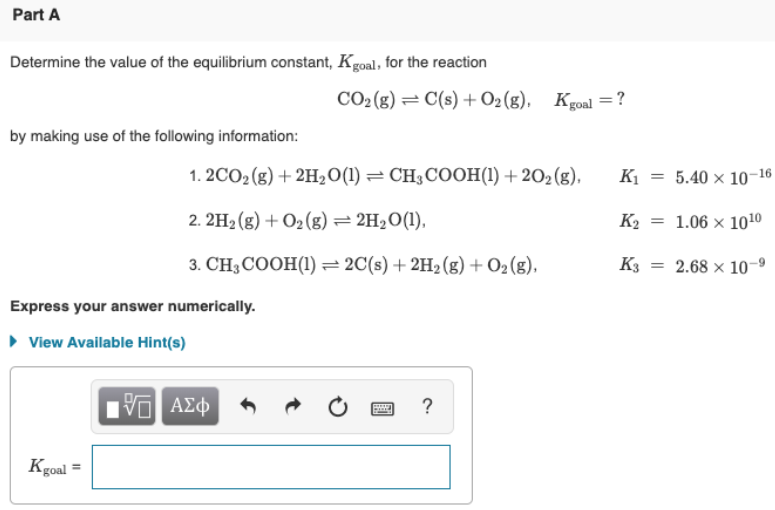# Determine the value of the equilibrium constant, Kgoal, for the reaction: CO2(g) ⇌ C(s) + O2(g), Kgoal=? by making use of the following information: 1. CO2(g)+2H2O(l) ⇌ CH3COOH(l)+2O2(g), K1 = 5.40×10^-16 2. 2H2(g) + O2(g) ⇌ 2H2O(l), K2 = 1.06×10^10 3. CH3COOH(l) ⇌ 2C(s) + 2H2(g) + O2(g), K3 = 2.68×10^-9 Express your answer numerically.